### 9.1.1.2 Multiobjective optimization

Suppose that there is a collection of cost functions, each of which evaluates an action. This leads to a generalization of Formulation 9.1 to multiobjective optimization.

Formulation 9..2 (Multiobjective Optimization)
1. A nonempty set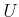called the action space. Each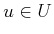is referred to as an action.
2. A vector-valued cost function of the form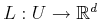for some integer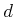. If desired,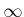may also be allowed for any of the cost components.

A version of this problem was considered in Section 7.7.2, which involved the optimal coordination of multiple robots. Two actions,and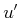, are called equivalent if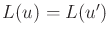. An actionis said to dominate an actionif they are not equivalent and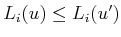for allsuch that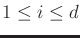. This defines a partial ordering,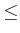, on the set of actions. Note that many actions may be incomparable. An action is called Pareto optimal if it is not dominated by any others. This means that it is minimal with respect to the partial ordering.

Example 9..1 (Simple Example of Pareto Optimality)   Suppose that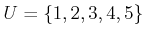and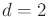. The costs are assigned as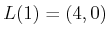,,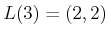,, and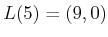. The actions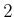,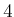, and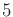can be eliminated because they are dominated by other actions. For example,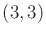is dominated by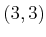; hence, action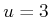is preferable to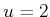. The remaining two actions,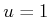and, are Pareto optimal.Based on this simple example, the notion of Pareto optimality seems mostly aimed at discarding dominated actions. Although there may be multiple Pareto-optimal solutions, it at least narrows downto a collection of the best alternatives.

Example 9..2 (Pennsylvania Turnpike)   Imagine driving across the state of Pennsylvania and being confronted with the Pennsylvania Turnpike, which is a toll highway that once posted threatening signs about speed limits and the according fines for speeding. Let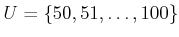represent possible integer speeds, expressed in miles per hour (mph). A posted sign indicates that the speeding fines are 1) $50 for being caught driving between 56 and 65 mph, 2)$100 for being caught between 66 and 75, 3) $200 between 76 and 85, and 4)$500 between 86 and 100. Beyond 100 mph, it is assumed that the penalty includes jail time, which is so severe that it will not be considered.

The two criteria for a driver are 1) the time to cross the state, and 2) the amount of money spent on tickets. It is assumed that you will be caught violating the speed limit. The goal is to minimize both. What are the resulting Pareto-optimal driving speeds? Compare driving 56 mph to driving 57 mph. Both cost the same amount of money, but driving 57 mph takes less time. Therefore, 57 mph dominates 56 mph. In fact, 65 mph dominates all speeds down to 56 mph because the cost is the same, and it reduces the time the most. Based on this argument, the Pareto-optimal driving speeds are 55, 65, 75, 85, and 100. It is up to the individual drivers to decide on the particular best action for them; however, it is clear that no speeds outside of the Pareto-optimal set are sensible.The following example illustrates the main frustration with Pareto optimality. Removing nondominated solutions may not be useful enough. In come cases, there may even be a continuum of Pareto-optimal solutions. Therefore, the Pareto-optimal concept is not always useful. Its value depends on the particular application.

Example 9..3 (A Continuum of Pareto-Optimal Solutions)   Let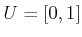and. Let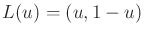. In this case, every element ofis Pareto optimal. This can be seen by noting that a slight reduction in one criterion causes an increase in the other. Thus, any two actions are incomparable.Steven M LaValle 2020-08-14